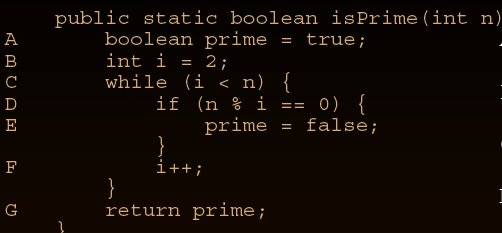What is McCabe’s Cyclomatic Complexity Number?

7
30
• 26
• 29
• 38
• 24
•
•
•
• 12
•
•
129
Shares

This material is highly important for those who are going for ISTQB training.
This is taken from ..Fenton and Pfleeger, Chapters 7 & 8.

If we talk about white box testing…this is a very important to understand what is the structure of the code..

The more “complex” the structure of code
◦ . . . the harder it is to understand
◦ . . . the more likely it will have a defect
◦ . . . the harder it will be to change
◦ . . . the longer it will take to produce
◦ . . . the more difficult it will be to reuse

Let us understand what is structure..
What is structure?
• control-flow — the sequence of instructions that are
executed
• data-flow — the creation and movement of data between
“components” of the code
• data organisation — the relationship of data items to each
other

let me go back to our engineering …where we have learnt the graph theory…
There are two types of graph mainly available..
1.Directed 2.Undirected.

Directed Graphs
• a mathematical structure (with an appealing visual
representation) for representing things that are related
• consists of vertices (or nodes, or points), connected by
edges (or line segments, or arcs).
Vertices: A, B, C, D, E, F
Edges: (A,C), (A,C), (A,E), (B,C), (B,F), (C,D), (D,A), (E,F),• Also: undirected graphs, rules restricting edges between
vertices, classification of vertices

now the question comes like what is flowgraph?
Flowgraphs
• Directed graphs (flowgraphs) can be used to model control
flow of a program
• vertex = statement, edge = (A,B) if control flows from
statement A to B
• properties of flowgraphs may provide information about
properties of the code

in the exam the question comes like find out the complexity of the below written code:

Example:

``````
public static boolean isPrime(int n) {
boolean prime = true;
int i = 2;
while (i < n)
{
if (n % i == 0)
{
prime = false;
}
i++;
}
return prime;
}
``````

let us put notation over here: as each of the line /statement is under flowgraph…i am putting A,B,C as the notation for the lines.Those will be my nodes where the controll of the pragramme will pass.the graph looks like:McCabe’s Cyclomatic Complexity Number (CCN)
• measures the number of linearly independent paths through
the flowgraph
• v(F) = e − n + 2, F the flowgraph of the code, n the number
of vertices, e the number of edges
• Intuition — the larger the CCN the “more complex” the code
• Various sources recommend a CCN of no more than 10-15
• Example: CCN = 8 − 7 + 2 = 3

try to understand this.and i will come back with several other examples.

Don't miss out!

Receive top technical news, lesson ideas, travel tips and more!

Give it a try. You can unsubscribe at any time.

• 26
• 29
• 38
• 24
•
•
•
• 12
•
•
129
Shares

1.Anonymous

Hello Ani !!!!!
Really thanks …. i m very confused in that topic but that article completely solve my problem.
tushar salgaonkar

2.Anonymous

if x<>=z
then stmt 2;
end

According to graph,i got 3 nodes and 2 edges.so,2-3+2=1.
I couldn't understand.Plz explain

3. Hei..we need to consider the else part as well
.
a…if x<>=z then
b…stmt 2
c…end if

Consider a case.. when x=z then it has to go end if i.e the c node

so there are two paths between A to c
1.a->b->c(if part)
2.a->c(else part)

so no of edges=3
No of nodes=3
so the cyclomatic complexity=e-N+2
3-3+2=2

look the second example above…see there is a if statement on D node…look even if there is no else part but that has been taken care.
same case will happen here also.

If you've still doubt, there is an easier equation that will make sense. Cyclomatic complexity can be explained in layman's terms as follows: every decision point in a method (i.e., an if, for, while, or case statement) is counted; additionally, one is added for the method's entry point, resulting in an integer-based measurement denoting a method's complexity.

so in this case there is only one if and only one entry point at node a.
so one if+one entry=Complexity=2

4. See my second exmaple where there is only one while and one if….
and also one entry point
so CC=1while+i if+1entry point
CC=3

5.Anonymous

Thanks Buddy!Now I understood.Thanks for Timely Response.

6.vinita

int i,j;
if(i>0 && j>0)
{
while(i>j)
{
if(i%2 && j%2)
printf("%dn" ,i);
else
printf("%dn" ,j);
i–;
}
}

The CC will be 4 is it correct?

7. Correct Vinita…
E=10
N=8
CC=E-N+2
CC=10-8+2
CC=4

let me know if you need any more help!!